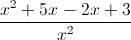# PSAT Math : How to subtract rational expressions with different denominators

## Example Questions

### Example Question #2 : Rational Expressions

Simplify.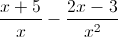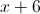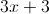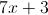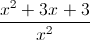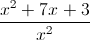Explanation:Determine an LCD (Least Common Denominator) betweenand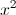.

LCD =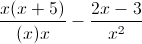Multiply the top and bottom of the first rational expression by, so that the denominator will be.

Distribute theto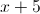.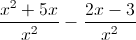Now you can subtract because both rational expressions have the same denominators.